# I need help with this Geometry exam before tomorrow!!

User Generated

Haxabjz5

Mathematics

Pearblossom

## Description

 ```Referring to the figure, complete the proportion (what is the numerator showing as a question mark): x ? — = —— 8 16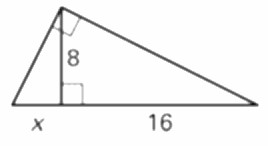``` 2 Answer: ``` Solve for x in problem #1. ``` 3 Answer: ``` Referring to the figure, complete the proportion (what is the denominator showing as a question mark): 4 x — = — x ?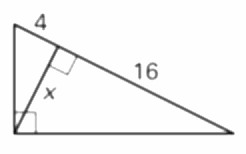``` 4 Answer: ``` Solve for x in problem #3. ``` 5 Answer: ``` Referring to the figure, complete the proportion (what is the numerator showing as a question mark): ? x — = — x 3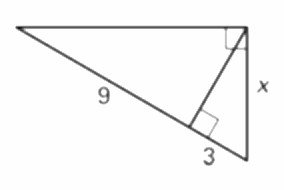``` 6 Answer: ``` Solve for x in problem #5. ``` 7 Answer: ``` Referring to the figure, on the right triangle shown, find the unknown side length. (If necessary, round to the nearest tenth.)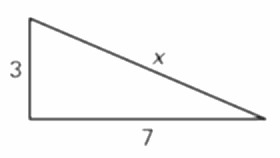``` 8 Answer: ``` Referring to the figure, on the right triangle shown, find the unknown side length. (If necessary, round to the neartest tenth.)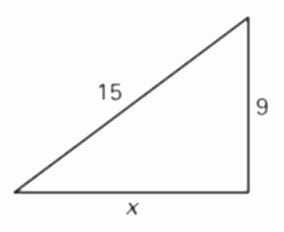``` 9 Answer: ``` Referring to the figure, on the right triangle shown, find the unknown side length. (If necessary, round to the neartest tenth.)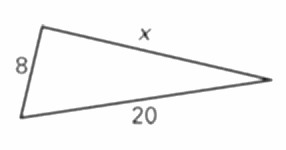``` 10 Answer: ``` Classify the triangle formed by the given side lengths: 6, 8, 10 a. right triangle b. acute triangle c. obtuse triangle d. not a triangle ``` 11 Answer: ``` Classify the triangle formed by the given side lengths: 3, 4, 6 a. right triangle b. acute triangle c. obtuse triangle d. not a triangle ``` 12 Answer: ``` Classify the triangle formed by the given side lengths: 6, 2, 5 a. right triangle b. acute triangle c. obtuse triangle d. not a triangle ``` 13 Answer: ``` Classify the triangle formed by the given side lengths: 5.4, 3.8, 6.5 a. right triangle b. acute triangle c. obtuse triangle d. not a triangle ``` 14 Answer: ``` Classify the triangle formed by the given side lengths: 1, 2, 3 a. right triangle b. acute triangle c. obtuse triangle d. not a triangle ``` 15 Answer: ``` Classify the triangle formed by the given side lengths: 1.6, 3.0, 3.4 a. right triangle b. acute triangle c. obtuse triangle d. not a triangle ``` 16 Answer: ``` Referring to the figure, find the value of x. (Hint: Use the Theorems about special right triangles and write the answer in simplest radical form.)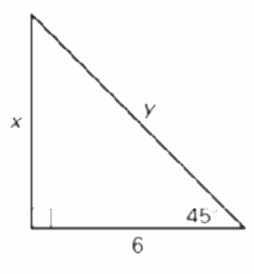``` 17 Answer: ``` Referring to the Fig. in Question #16, find the value of y. (Hint: Use the Theorems about special right triangles and write the answer in simplest radical form.) ``` 18 Answer: ``` Referring to the figure, find the value of a. (Hint: Use the Theorems about special right triangles and write the answer in simplest radical form.)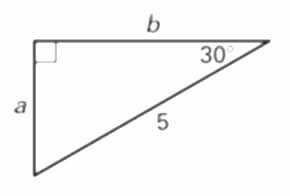``` 19 Answer: ``` Referring to the Fig. in Question #18, find the value of b. (Hint: Use the Theorems about special right triangles and write the answer in simplest radical form.) ``` 20 Answer: ``` Referring to the figure, find the value of m. (Hint: Use the Theorems about special right triangles and write the answer in simplest radical form.)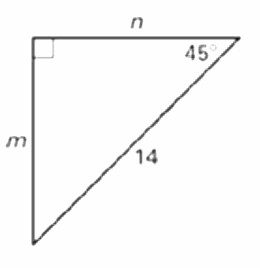``` 21 Answer: ``` Referring to the Fig. in Question #20, find the value of n. (Hint: Use the Theorems about special right triangles and write the answer in simplest radical form.) ``` 22 Answer: ``` Referring to the figure, find the sine of angle A. Express the value as a decimal rounded to four places.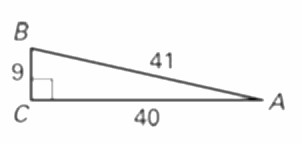``` 23 Answer: ``` Referring to the Fig. in Question #22, find the cosine of angle A. Express the value as a decimal rounded to four places. ``` 24 Answer: ``` Referring to the Fig. in Question #22, find the tangent of angle A. Express the value as a decimal rounded to four places. ``` 25 Answer: ``` Referring to the figure, find the sine of angle A. Express the value as a decimal rounded to four places.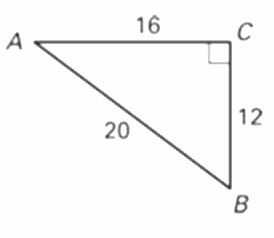``` 26 Answer: ``` Referring to the Fig. in Question #25, find the cosine of angle A. Express the value as a decimal rounded to four places. ``` 27 Answer: ``` Referring to the Fig. in Question #25, find the tangent of angle A. Express the value as a decimal rounded to four places. ``` 28 Answer: ``` Referring to the figure, find the sine of angle A. Express the value as a decimal rounded to four places.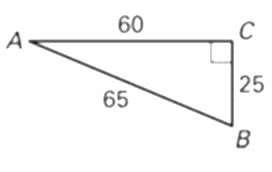``` 29 Answer: ``` Referring to the Fig. in Question #28, find the cosine of angle A. Express the value as a decimal rounded to four places. ``` 30 Answer: ``` Referring to the Fig. in Question #28, find the tangent of angle A. Express the value as a decimal rounded to four places. ``` 31 Answer: ``` Referring to the figure, use trigonometric ratios to find the value of x. Round decimals to the nearest tenth.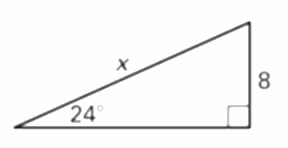``` 32 Answer: ``` Referring to the figure, use trigonometric ratios to find the value of x. Round decimals to the nearest tenth.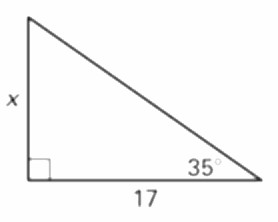``` 33 Answer: ``` The distance of the base of a ladder from the wall it leans against should be at least 1/4 of the ladder's total length. Suppose a 10 foot ladder is placed according to these guidelines. Give the minimum distance of the base of the ladder from the wall. ``` 34 Answer: ``` Referring to the ladder in problem 33, how far up the wall will the ladder reach?```User generated content is uploaded by users for the purposes of learning and should be used following Studypool's honor code & terms of service.Buddy, there you have it. Thank you
Hello buddy, here are the edited solution. Please go through it and let me know if there is any need for further edit. Thank you

7. 32 + 72 = x2

x =√(9+49)
x= √58
x =7 .6

9...### ReviewAnonymous
Excellent resource! Really helped me get the gist of things.Studypool4.7Trustpilot4.5Sitejabber4.4